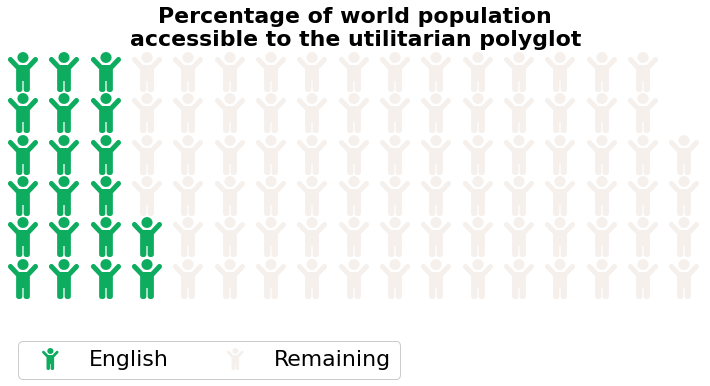Utilitarian Polyglot: What languages do you need to speak with the most people?¶Libraries¶

Let's import libraries for analysind and visualising data.

In :
import pandas as pd
import seaborn as sns
import matplotlib.pyplot as plt
from pywaffle import Waffle
import folium

English-speaking populations in various countries¶

This data originates from Wikipedia's List of countries by English-speaking population. Some countries have been added (see column Source) and some names of countries have been changed.

In :
'proportion_english_speaking.csv'
)
In :
Out:
Country Total population Total English speakers Percentage Source
0 Algeria 35954000 2516780 0.0700 https://en.wikipedia.org/wiki/List_of_countrie...
1 American Samoa 57345 45933 0.8010 https://en.wikipedia.org/wiki/List_of_countrie...
2 Andorra 81222 17868 0.2200 https://en.wikipedia.org/wiki/List_of_countrie...
3 Anguilla 13000 12000 0.9231 https://en.wikipedia.org/wiki/List_of_countrie...
4 Antigua and Barbuda 85000 68000 0.8000 https://en.wikipedia.org/wiki/List_of_countrie...
In :
total_english = proportion_english_speaking['Total English speakers'].sum()
total_world = 7800000000
print(
'''
Total number of English-speakers:\t\t{:,}
As a percentage of world population:\t{:.0%}
'''.format(
total_english,
total_english / total_world
)
)
Total number of English-speakers:		1,521,817,350
As a percentage of world population:	20%

In :
accessible = {
'English': total_english / total_world * 100,
'Remaining': (total_world - total_english) / total_world * 100
}

fig = plt.figure(
FigureClass=Waffle,
rows=6,
values=accessible,
colors=["#0dac5f", "#f5f0ec"],
title={
'label': 'Percentage of world population\naccessible to the utilitarian polyglot',
'loc': 'center',
'fontsize': 22,
'fontweight': 'bold'
},
legend={
'loc': 'lower left',
'bbox_to_anchor': (0, -0.4),
'ncol': len(accessible),
'framealpha': 1,
'fontsize': 22
},
icons='child',
font_size=40,
figsize=(10, 5),
icon_legend=True
)Let's plot the proportion of English-speaking populations by country on a map. The map comes from here. The names of some countries have been changed to fit with other data.

In :
with open('world-countries.json') as geojson:

m = folium.Map(
location=[30, 0],
tiles='CartoDB Positron No Labels',
zoom_start=2,
min_zoom=2,
zoom_control=False,
min_lat=-60,
max_lat=80,
max_bounds=True,
)

map = folium.Choropleth(
geo_data=maps,
name='choropleth',
data=proportion_english_speaking,
columns=['Country', 'Percentage'],
key_on='feature.properties.name',
fill_color='RdYlGn',
nan_fill_color='black',
nan_fill_opacity=0.2,
line_color='white'

folium.GeoJsonTooltip(
fields=['name'],
labels=False

folium.Marker(
location=[-40, -40],
icon=folium.DivIcon(
html='''
<div style="
background-color: rgba(242, 242, 242, 0.5);
box-shadow: 4px 4px 8px 0 rgba(0, 0, 0, 0.2), 6px 6px 20px 0 rgba(0, 0, 0, 0.19);
border: 1px solid black;
display: inline-block;
font-size: 20px;
">
<span>
<strong>Percentage of English-speaking population</strong>
<br /><strong>by country</strong>
</span>
</div>
''',
icon_size=(1000, 0),
icon_anchor=(0, 0),
)

display(m)
Make this Notebook Trusted to load map: File -> Trust Notebook

Let's see how much data we're missing here. The population numbers come from Wikipedia's List of countries by population. The names of some countries have been changed to fit with other data.

In :
'population.csv'
)

population.set_index('Country', inplace=True)

Out:
Population
Country
China 1405660160
India 1370601758
United States 330781533
Indonesia 269603400
Pakistan 220892331
In :
# getting a list of all countries
countries = []

for i in maps['features']:
countries.append(i['properties']['name'])

# populating (ha) the list of countries we don't have data on
population_index = []

for i in sorted(set(countries).difference(set(proportion_english_speaking['Country']))):
if i in population.index:
population_index.append(i)

unknown = population.loc[population_index].sort_values('Population', ascending=False)

print(
'''
No data - number of countries:\t{}

No data - total population:\t\t{:,}
'''.format(
len(unknown), unknown['Population'].sum()
)
)

unknown
No data - number of countries:	73

No data - total population:		799,176,501

Out:
Population
Country
Sudan 43051890
Uzbekistan 34568514
Saudi Arabia 34218169
Afghanistan 32890171
Peru 32625948
... ...
Western Sahara 597000
The Bahamas 389410
Northern Cyprus 351965
Greenland 56367
Falkland Islands 3198

73 rows × 1 columns